Get instant live expert help with Excel or Google Sheets“My Excelchat expert helped me in less than 20 minutes, saving me what would have been 5 hours of work!”

#### Post your problem and you’ll get expert help in seconds.

Your message must be at least 40 characters
Our professional experts are available now. Your privacy is guaranteed.

# What is the Difference Between SUMIF vs. SUMIFS vs. SUMFPRODUCT?

Excel has some of the most powerful functions for us in order to make our work much easier.

Functions under discussion are considered as advanced excel functions.

In this tutorial, we will learn the difference between the following functions as follow:

1. SUMIF
2. SUMIFS
3. SUMPRODUCT

## SUMIF

SUMIF function help us to add different values based on one condition/criteria in an array.

The formula of the SUMIF is as follow:

`=SUMIF(range, criteria, [sum range])`

Our data set is as follow: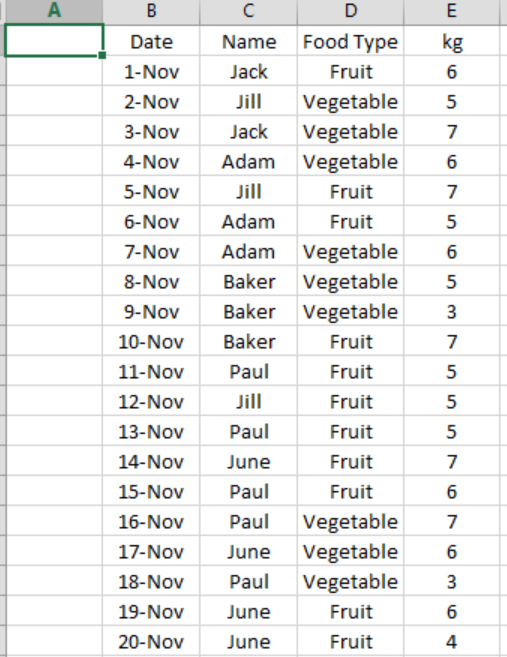Figure 1: Data Set

Based on our data set we want to know how much kg of vegetable & fruits “June” bought over the time.

In cell “H2”, write formula SUMIF, in “range” portion select Column “C” (Name), criteria will be “June” & in “Sum Range” portion of formula select column “E” (kg).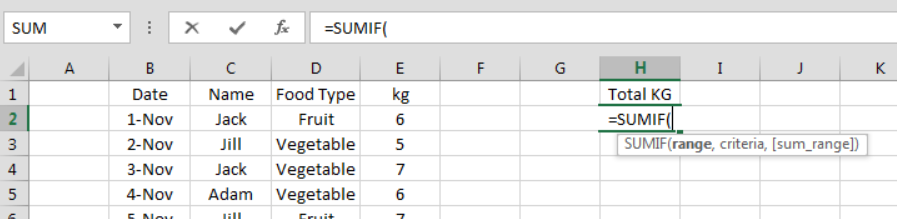Figure 2: SumIf Formula

Below mentioned screenshot will show the formula & cell references: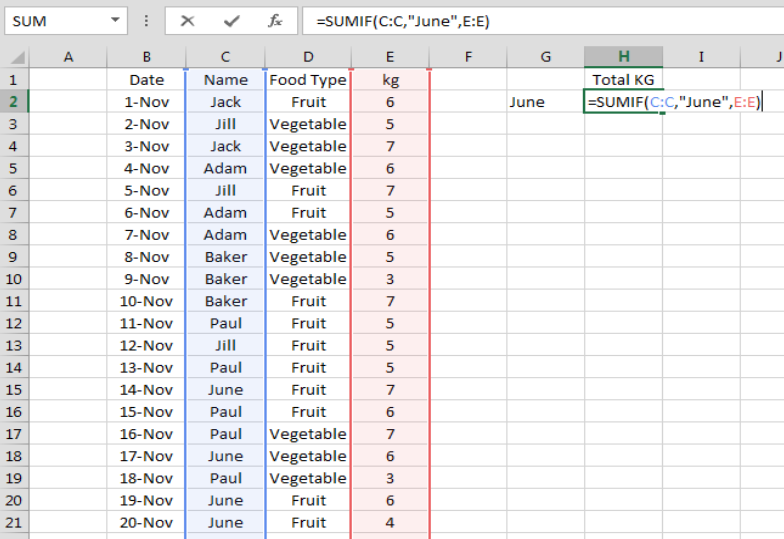Figure 3: Selecting Formula Range

After entering the formula, the result is as follow: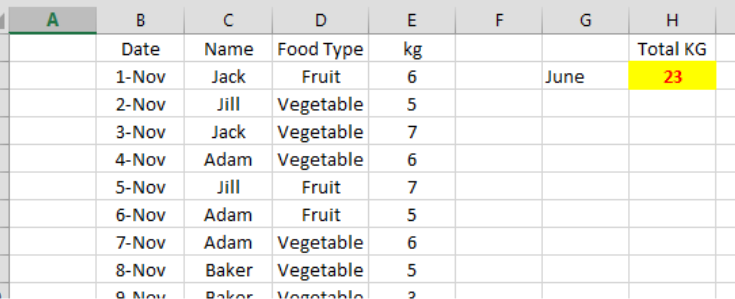Figure 4: Completing Formula & Result

## SUMIFS

SUMIFS function help us to add different values based on multiple condition/criteria in an array.

The formula of the SUMIFS is as follow:

`=SUMIF(sum_range, criteria_range1, criteria1, [criteria_range2, criteria2]……. )`

In SUMIFS, criteria2 and onward are optional. Now we continue with the same data set.

Now we want to know how much kg “June” bought in Food Type “Fruit”.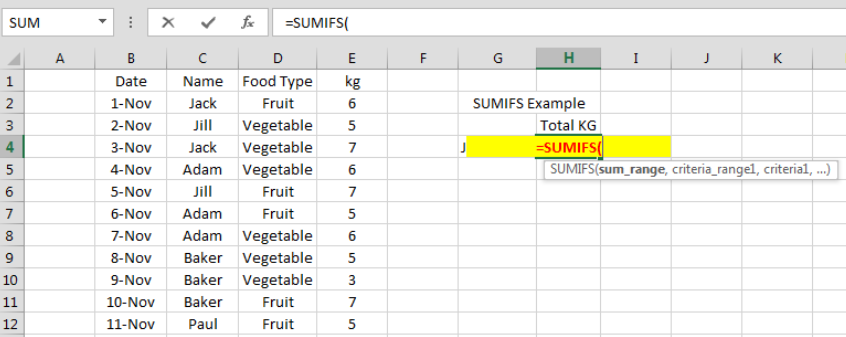Figure 5: SumIFs Formula

Here our sum range will be column “E” i.e, Kg, criteria 1 will be “June” and criteria 2 will be Food Type “Fruit”. The screenshot is as follow: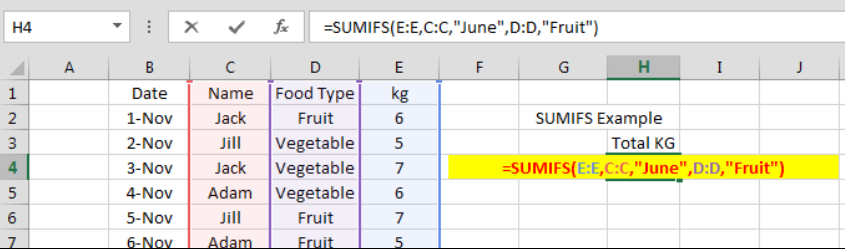Figure 6: SumIFs Formula Selecting Range

And the final result is as follow: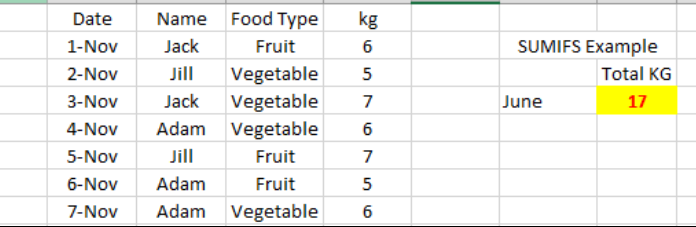Figure 7: SumIFs Formula Result

## SUMPRODUCT

The SUMPRODUCT function multiples ranges or arrays together and return the sum of products. SUMPRODUCT is a very versatile function.

`=SUMPRODUCT(array1, [array2], ….)`

For the sake of SUMPRODUCT, we consider price of vegetable & fruit.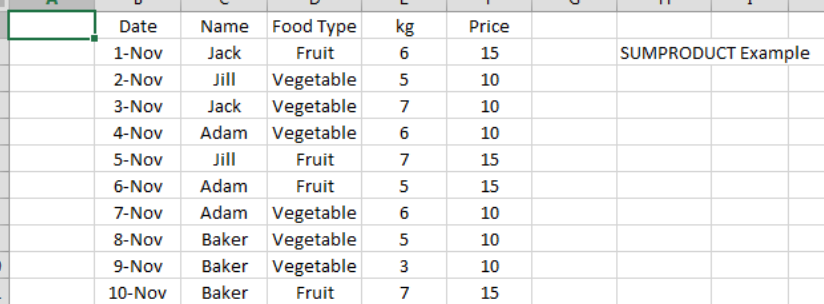Figure 8: SUMPRODUCT data

Now we want to calculate total sale of both vegetables & fruit.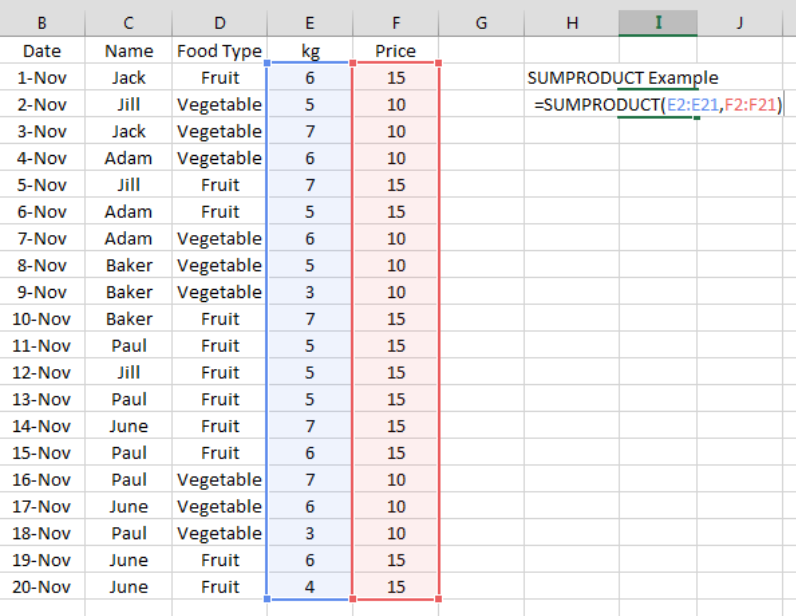Figure 9: SUMPRODUCT Formula Selecting Range

Here SUMPRODUCT function is first multiplying values of array1 with array2 and then summing the product value of array1 & array2.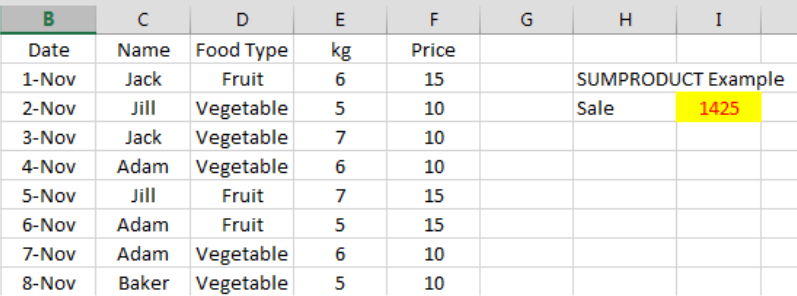Figure 10: SUMPRODUCT Formula Result

Most of the time, the problem you will need to solve will be more complex than a simple application of a formula or function. If you want to save hours of research and frustration, try our live Excelchat service! Our Excel Experts are available 24/7 to answer any Excel question you may have. We guarantee a connection within 30 seconds and a customized solution within 20 minutes.

Solution examplesamending a formula so that there is another criteria and if it does not meet this criteria then we stick with the original criteria
Solved by A. B. in 40 minsI am looking for a formula to SUM column L, if Column A matched the relevant date and column C matches the name
Solved by Z. Y. in 16 minsI am trying to utilize a complicated sumif or sumifs formula that can pull a total amount from a date and a drop down box from another tab.
Solved by E. B. in 15 minsI have a spreadsheet that totals invoices. A bunch of cells at the bottom of the spreadsheet calculates totals based on which department the invoice is charged to. I'd like to add a function where it also considers the date (so if it's an invoice in January, it goes to a January total, February, etc.). But I can't figure out how to write it properly...
Solved by K. J. in 27 minsIf I want to write a formula that states" "If this name shows up K24, and this word shows up in J24 pull this number from M24" I can creat that formula - however I need to drag this down so it pulls from row 24 - 60 - is there way to do this?
Solved by F. D. in 40 mins